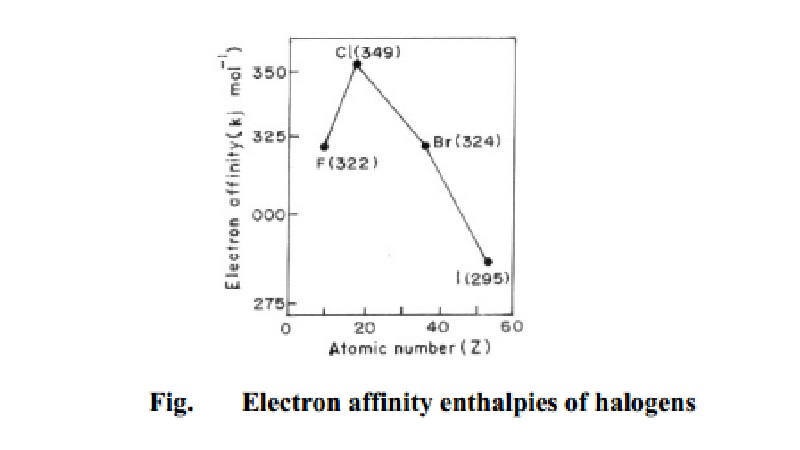Home | | Chemistry | Electron affinity

# Electron affinityIn modern terminology, electron affinity is known as the electron gain enthalpy. Electron gain enthalpy is the amount of energy released when an isolated gaseous atom accepts an electron to form a monovalent gaseous anion.

Electron affinity

In modern terminology, electron affinity is known as the electron gain enthalpy. Electron gain enthalpy is the amount of energy released when an isolated gaseous atom accepts an electron to form a monovalent gaseous anion.

Atom (g) + Electron -> Anion (g) + energy

Example, Cl (g) + e--> Cl- (g) + EA

If an atom has high tendency to accept an electron, large energy will be released. Consequently, electron gain enthalpy will be high. On the other hand if an atom has less tendency to accept the electron small amount of energy will be released, leading to small value of electron gain enthalpy. The values of electron gain enthalpy are expressed either in electron volt per atom or kilo joules per mole of atoms. For example, electron gain enthalpy of

F  = 322 kJ mol-1

Cl = 349 kJ mol-1

Br = 324 kJ mol-1 and

I  =295 kJ mol-1

Halogens (elements of group 17) can take up an electron to acquire the stable noble gas configuration. Their values for electron gain enthalpy are thus very high. Electron gain enthalpy values for the halogens are as in Fig. 4.5.

Electron gain enthalpies generally decrease on moving down the group. This is expected on account of the increase in size of atoms, the effective nuclear attraction for electrons decreases. As a result, there is less tendency to attract additional electrons with an increase in atomic number down the group.

From the electron gain enthalpy data of halogens it is clear that, contrary to expectation, the electron gain enthalpy of fluorine is lower than that of chlorine. This is because the fluorine atom has a very compact electronic shell due to its small size. The compactness of the fluorine shell results in electron repulsion whenever an electron is introduced into its 2p-orbital. This is why its electron gain enthalpy is less than the expected value. In Cl atom, 3p-orbitals are not as compact as the 2p-orbitals in fluorine atom. The incoming electron is more readily accepted by the chlorine atom because of weaker electron-electron repulsion. The electron gain enthalpy of chlorine is, therefore, higher than that of fluorine.

In the case of noble gases, the outer s-and p-orbitals are completely filled. No more electrons can be accommodated in these orbitals. Noble gases, therefore, show no tendency to accept electrons. Their electron gain enthalpies are zero.

Electron gain enthalpies g The second electron gain enthalpy refers to a process in which the electron is added to a negative ion. For example:

O- (g) + e- -> O2- (g)

Since a negative ion O- and an electron repel each other, energy is required and not released by the process. Therefore the second electron gain enthalpy is negative in this case.

Factors influencing the magnitude of electron affinity

The magnitude of EA is influenced by a number of factors such as

(i) Atomic size; (ii) Effective nuclear charge; and (iii) Screening effect by inner electrons.

Example

Which of the following will have the most negative electron gain enthalpy and which has the least negative? P, S, Cl, F.

Solution

Electron gain enthalpy generally becomes more negative across a period as we move from left to right. Within a group, electron gain enthalpy becomes less negative down a group. However, adding an electron to the 2p orbital leads to greater repulsion than adding an electron to the larger 3p orbital. Hence the element with most negative electron gain enthalpy is chlorine; the one with the least negative electron gain enthalpy is phosphorus.

enerally increase as we move across a period from left to right. This is due to the increase in the nuclear charge, which results in greater attraction for electrons.

Study Material, Lecturing Notes, Assignment, Reference, Wiki description explanation, brief detail
11th 12th std standard Class Organic Inorganic Physical Chemistry Higher secondary school College Notes : Electron affinity |